# r-process enhanced stars¶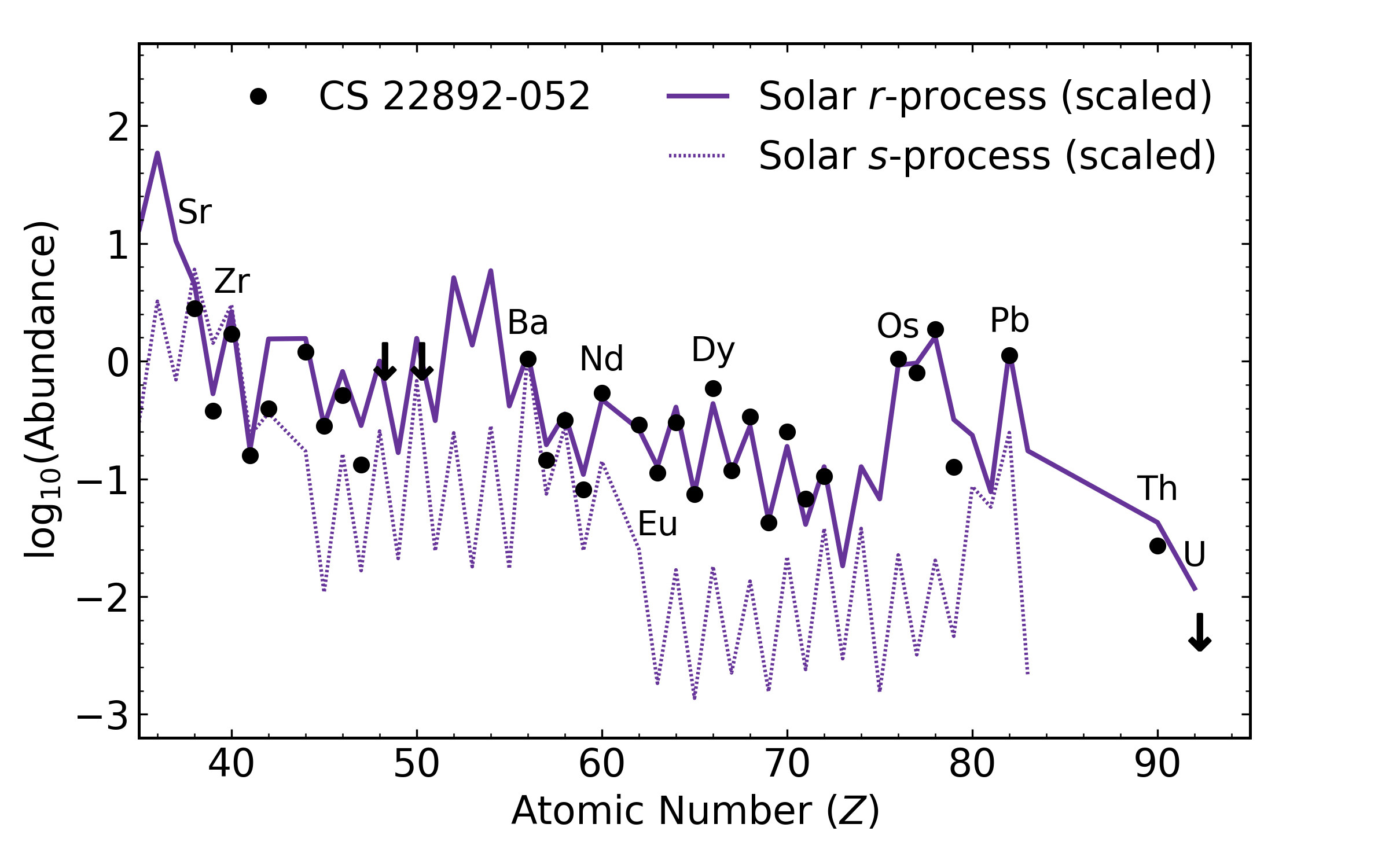## from data in McWilliam+ (1995), Sneden+ (2003)¶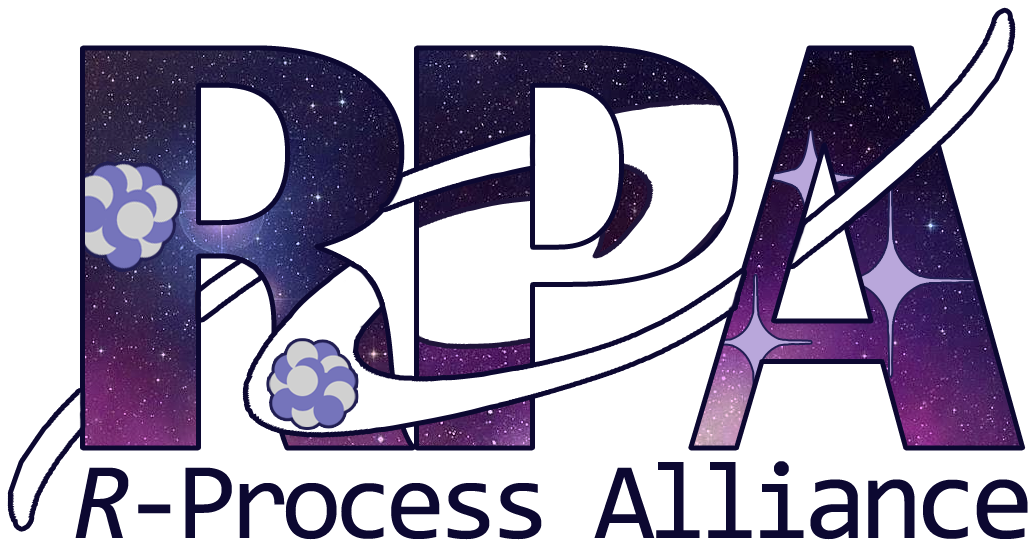# Uranium in RAVE J203843.2-002333¶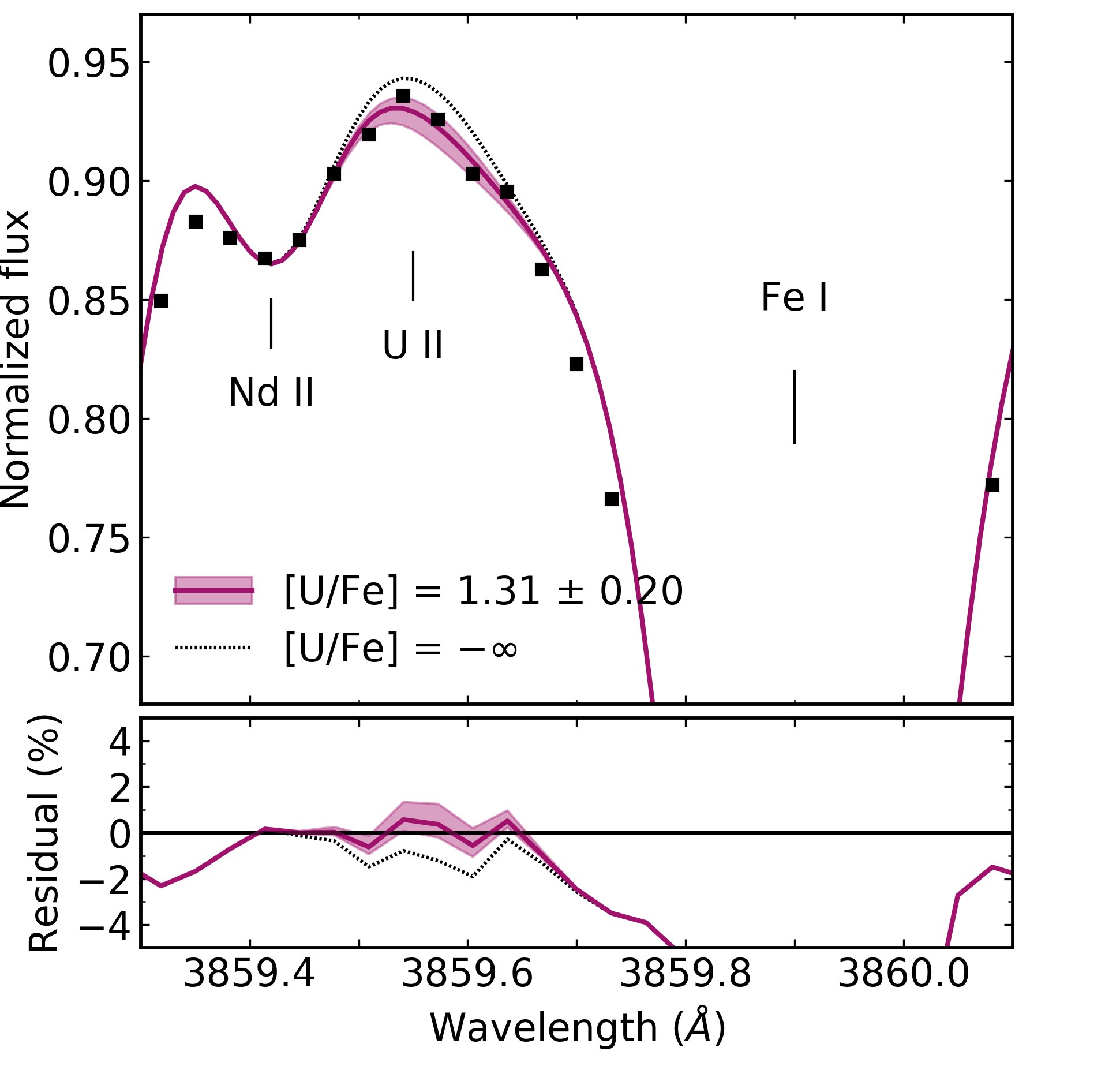# Uranium in J0954+5246¶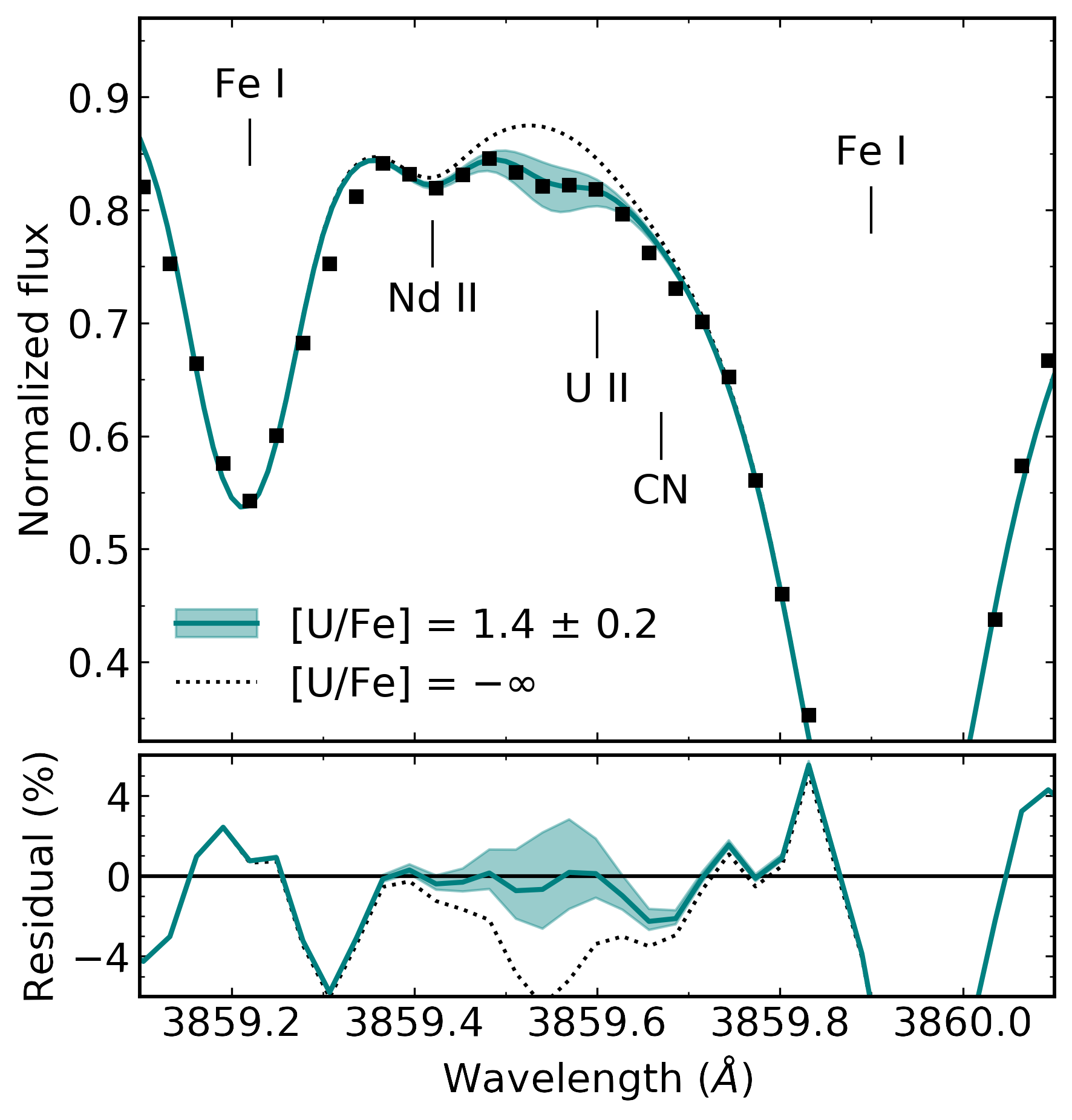# The actinide boost and J0954+5246¶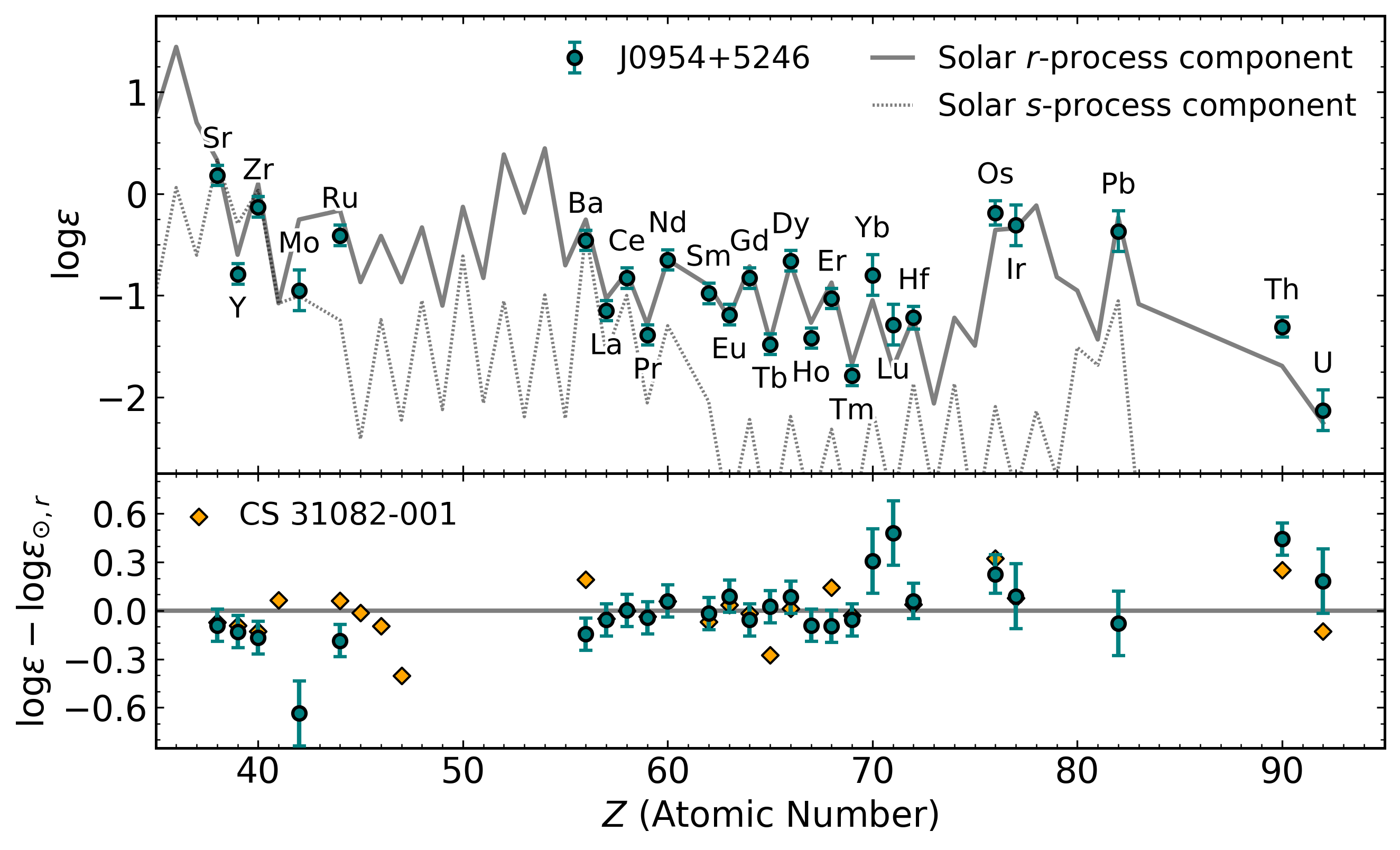# Ages and cosmochronometry¶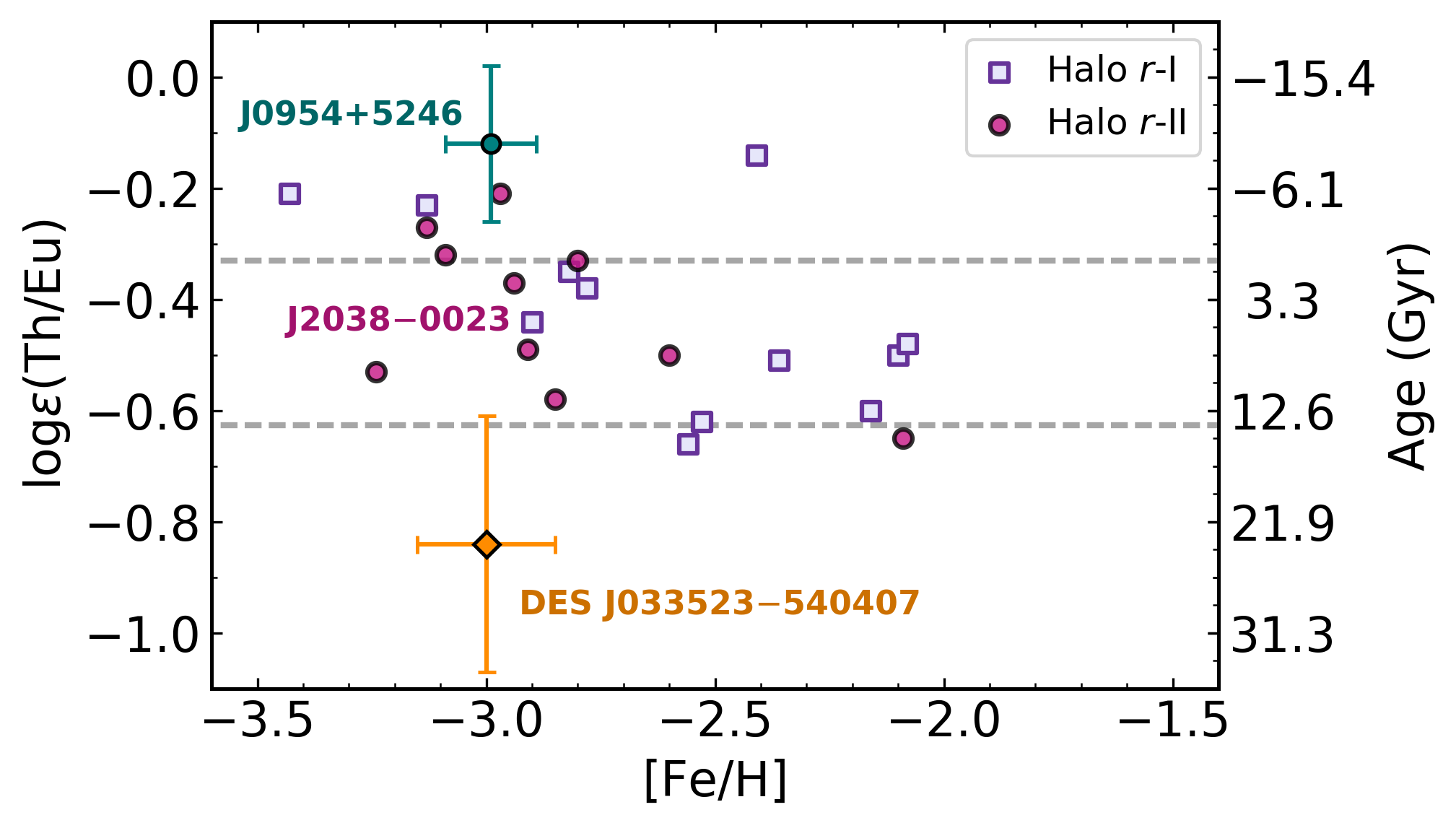# Ages and cosmochronometry¶

$$t = 46.67~\text{Gyr} \left[\log\epsilon\left(\text{Th/Eu}\right)_0 - \log\epsilon\left(\text{Th/Eu}\right)_{\text{obs}}\right]$$
$$t = 14.84~\text{Gyr} \left[\log\epsilon\left(\text{U/Eu}\right)_0 - \log\epsilon\left(\text{U/Eu}\right)_{\text{obs}}\right]$$
$$t = 21.80~\text{Gyr} \left[\log\epsilon\left(\text{U/Th}\right)_0 - \log\epsilon\left(\text{U/Th}\right)_{\text{obs}}\right]$$

# Can varying levels of neutron richness in a NSM account for the actinide boost?M. Mumpower and T. Sprouse

Low-entropy dynamical (tidal) ejecta of a NSM
(Korobkin+ 2012; Rosswog+ 2013)

Vary the initial electron fraction: $Y_e=0.005 - 0.250$

$Y_e = \left[1+(n/p)\right]^{-1}$

# Actinide and lanthanide production¶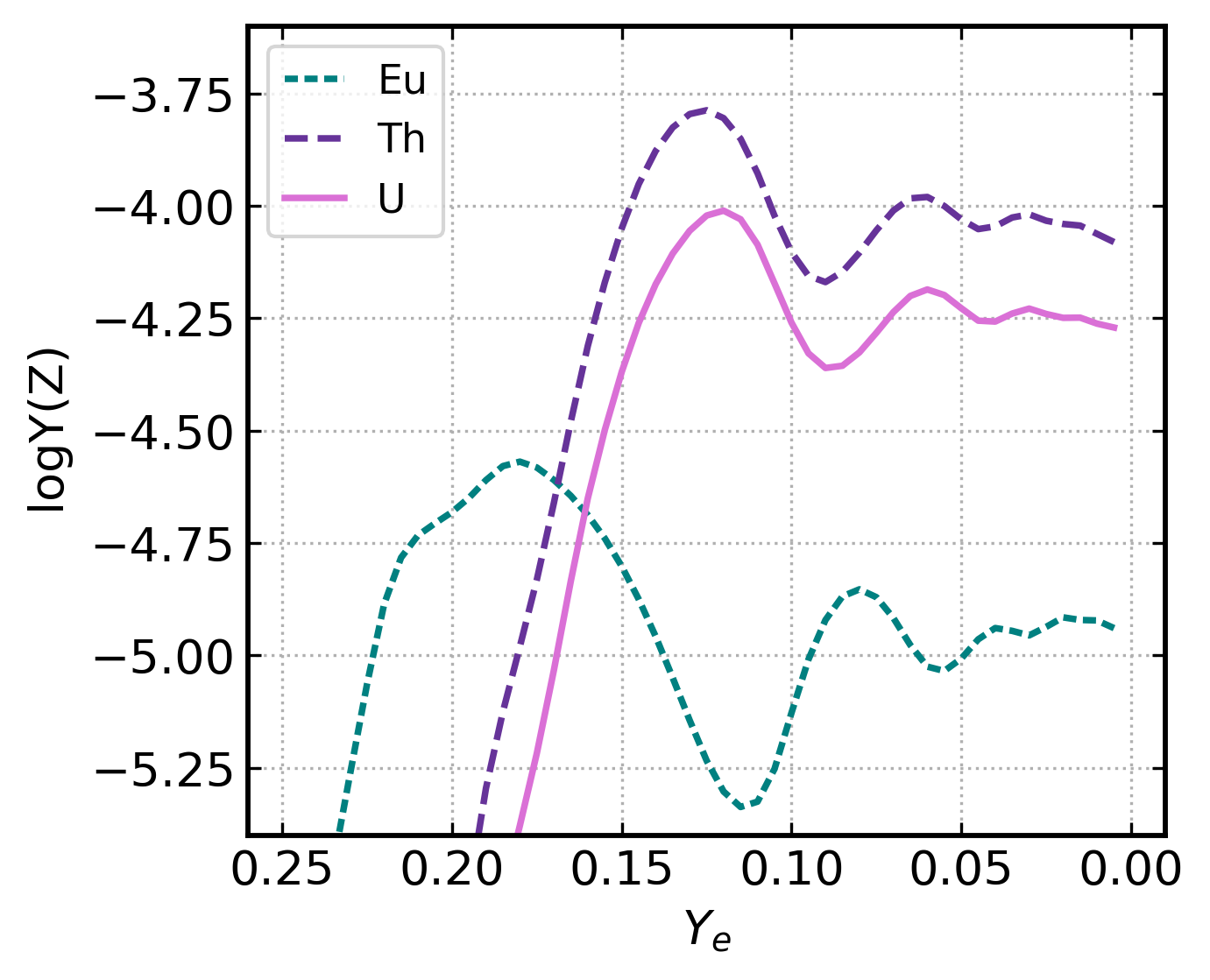# Ages and cosmochronometry¶

$$t = 46.67~\text{Gyr} \left[\log\epsilon\left(\text{Th/Eu}\right)_0 - \log\epsilon\left(\text{Th/Eu}\right)_{\text{obs}}\right]$$
$$t = 14.84~\text{Gyr} \left[\log\epsilon\left(\text{U/Eu}\right)_0 - \log\epsilon\left(\text{U/Eu}\right)_{\text{obs}}\right]$$
$$t = 21.80~\text{Gyr} \left[\log\epsilon\left(\text{U/Th}\right)_0 - \log\epsilon\left(\text{U/Th}\right)_{\text{obs}}\right]$$

# The age of J0954+5246¶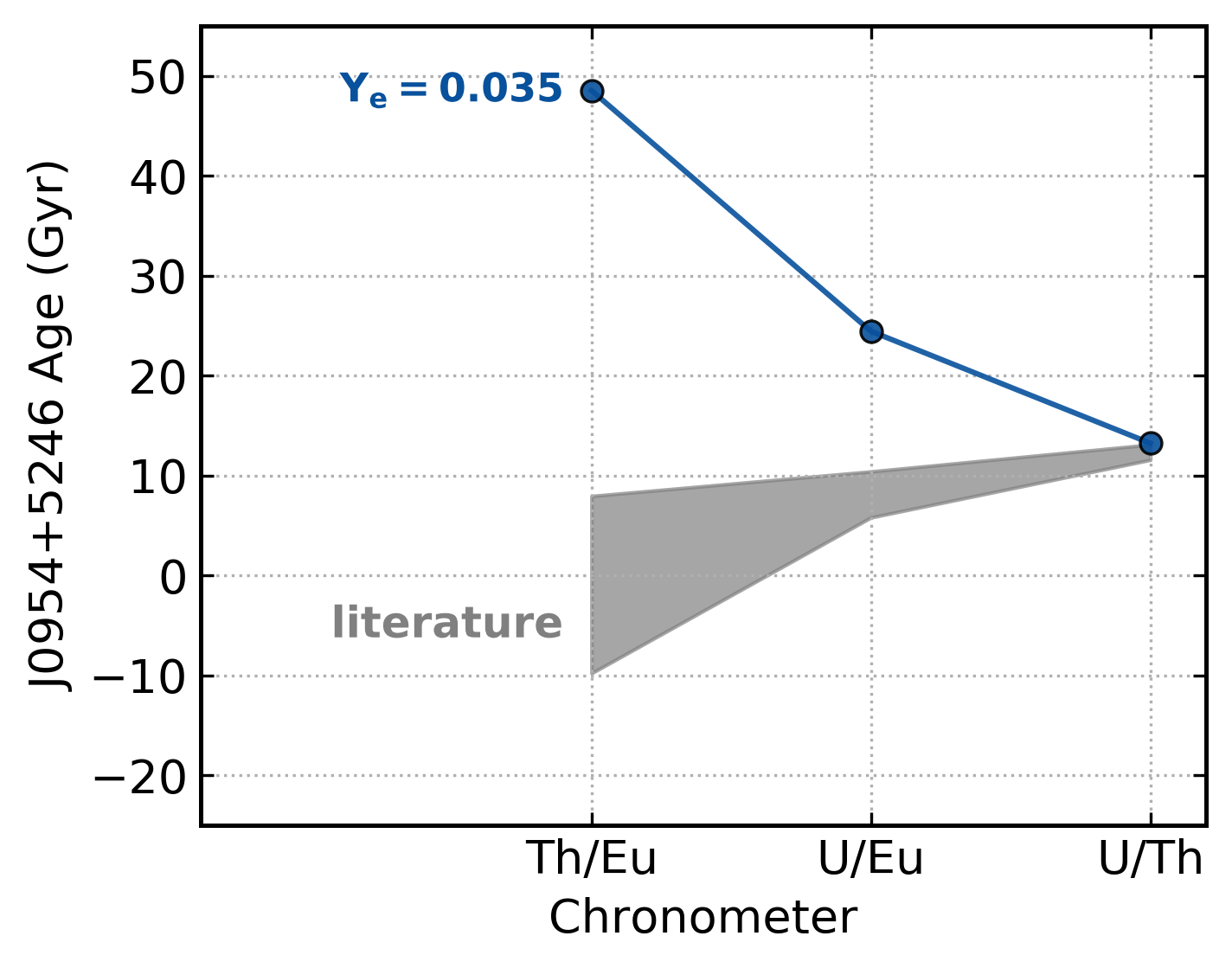# Nuclear physics variations¶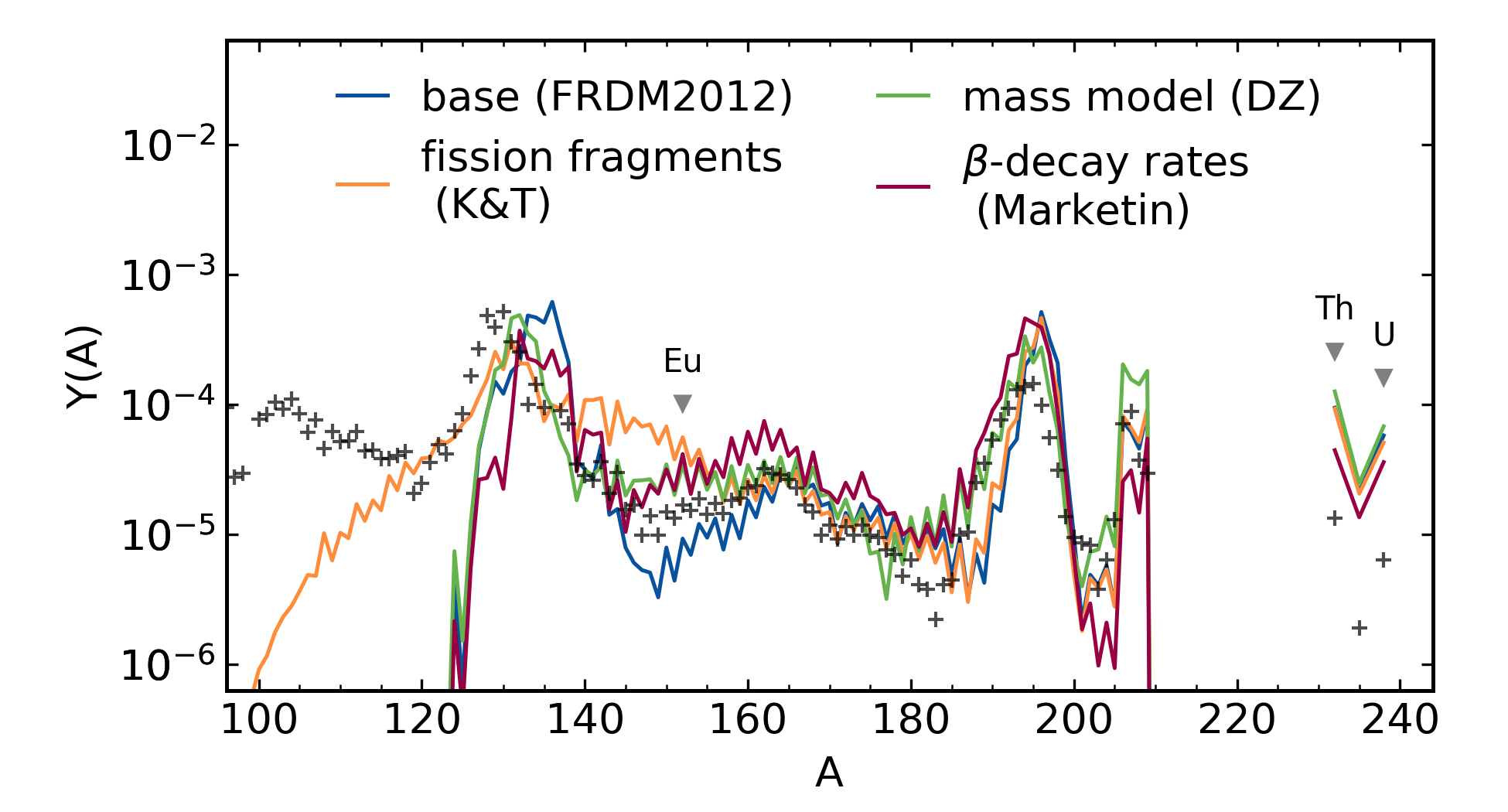# The age of J0954+5246¶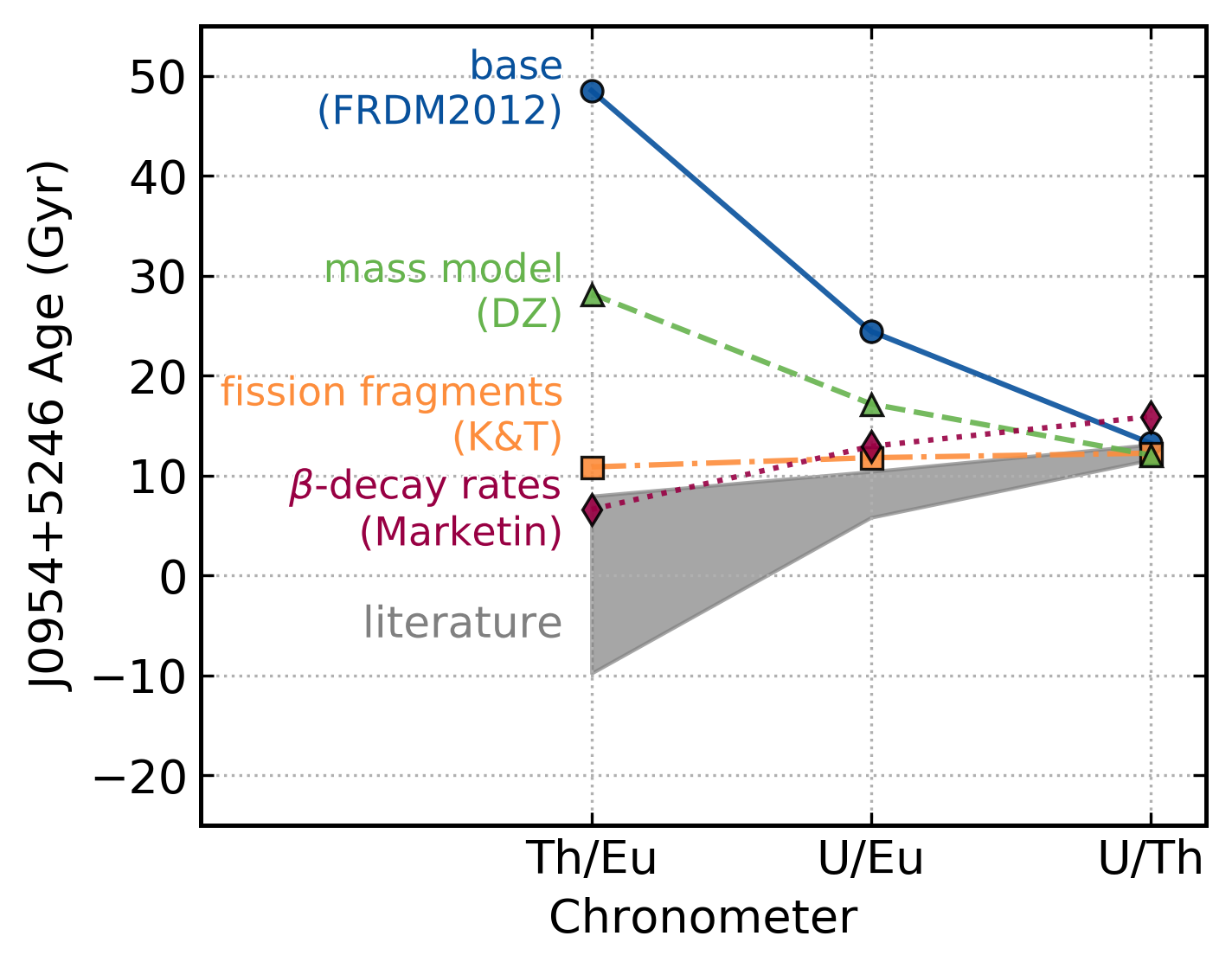# Actinides are currently not observed at such high levels

Need a method to dilute the actinides to reduce the Th/Eu production ratio

# Actinide-Dilution model¶

## Distribution of $Y_e$¶

Tidal ejecta
($Y_e\approx 0.16$, Bovard+ 2017)

Disk wind
($Y_e\approx 0.22$; Lippuner+ 2017)

$m_{\rm wind}/m_{\rm dyn}= 3$
from estimates of GW170817
(Rosswog+ 2017; Tanaka+ 2017)

# Actinide-Dilution model¶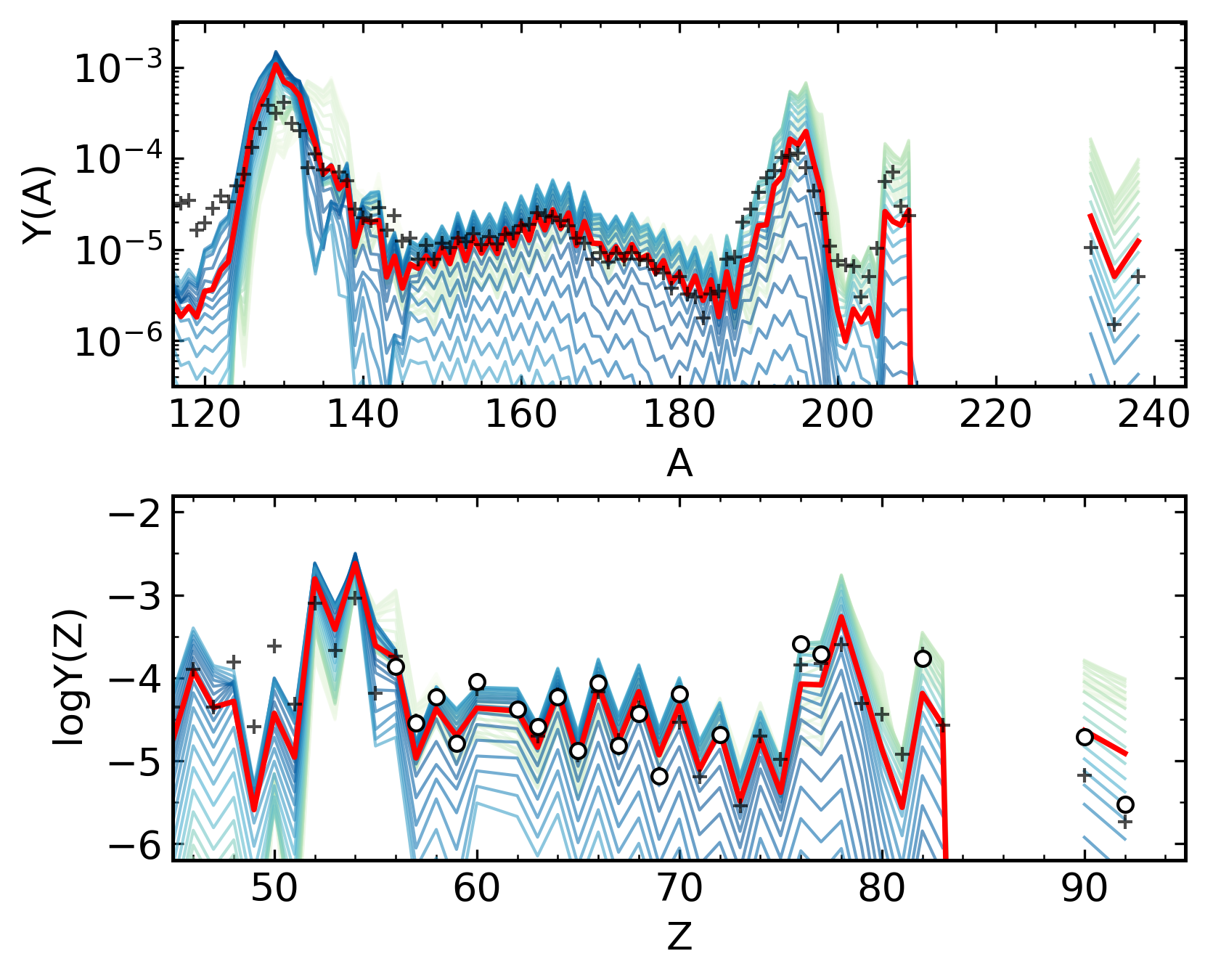# Ages¶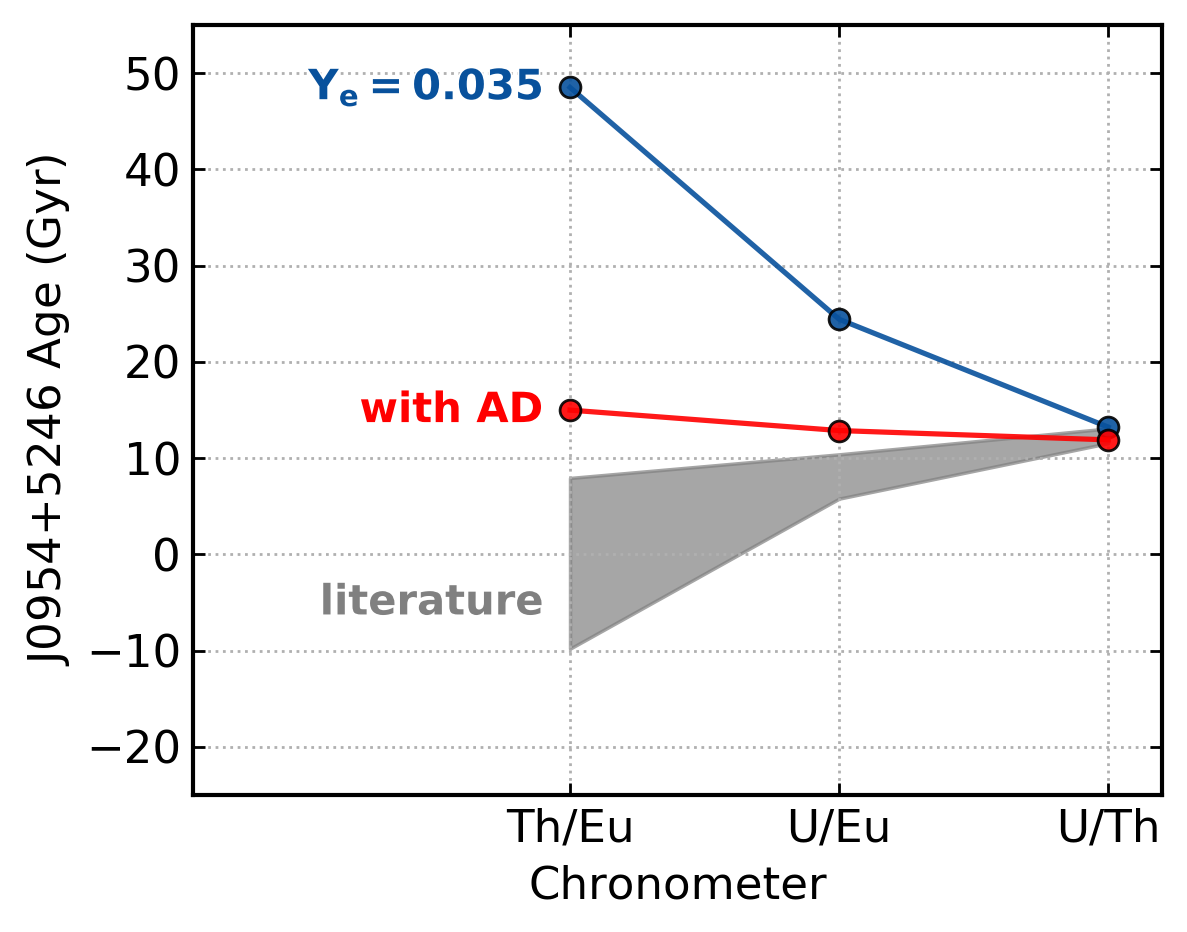# Ages¶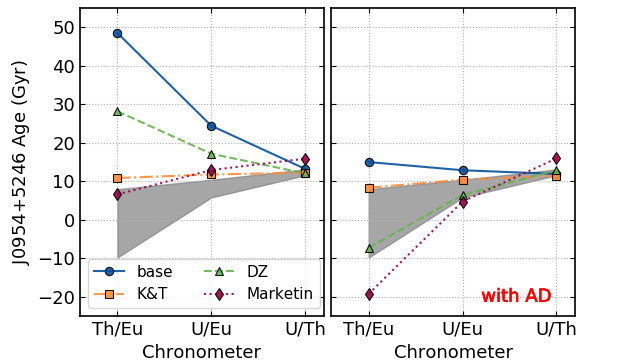## Holmbeck+ (2019)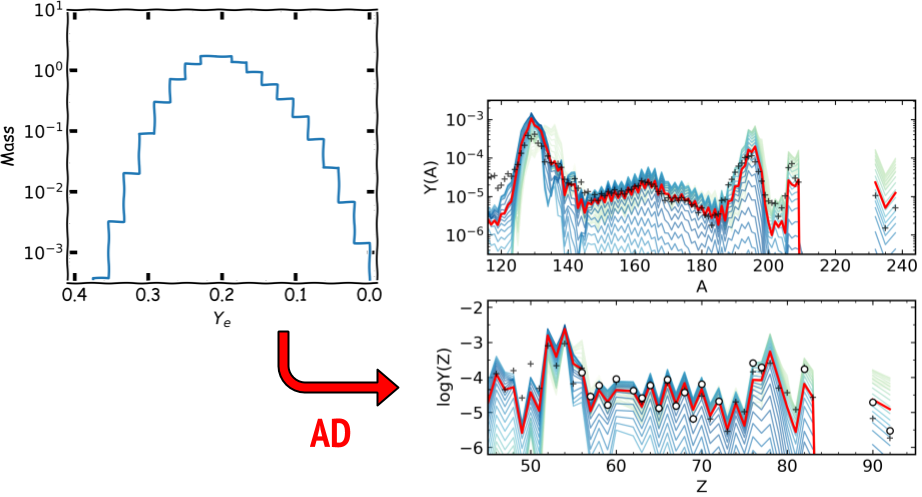# Can we go backwards?¶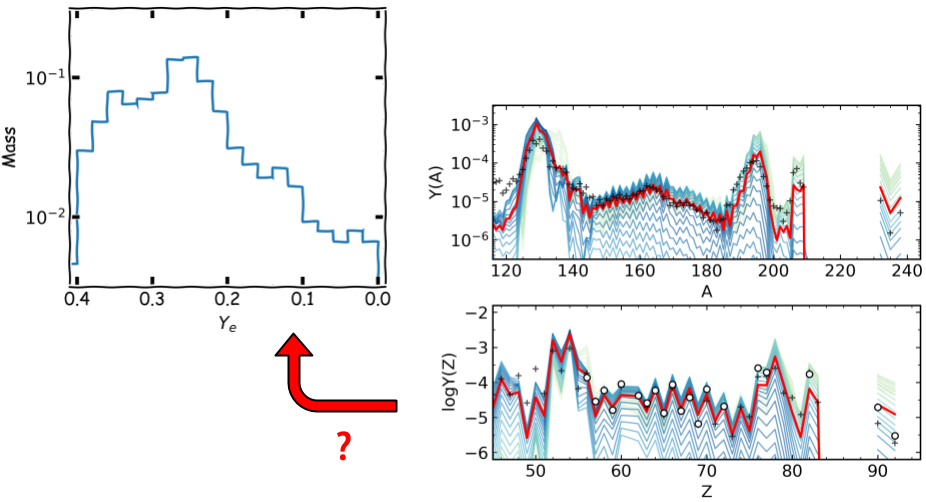# Reticulum II¶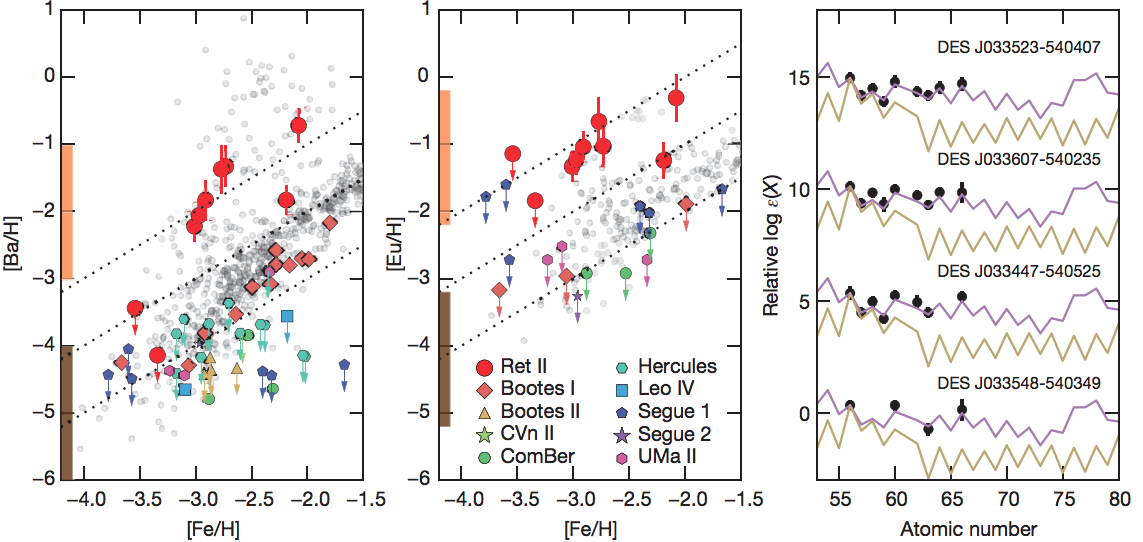# Groups of r-process enhanced stars¶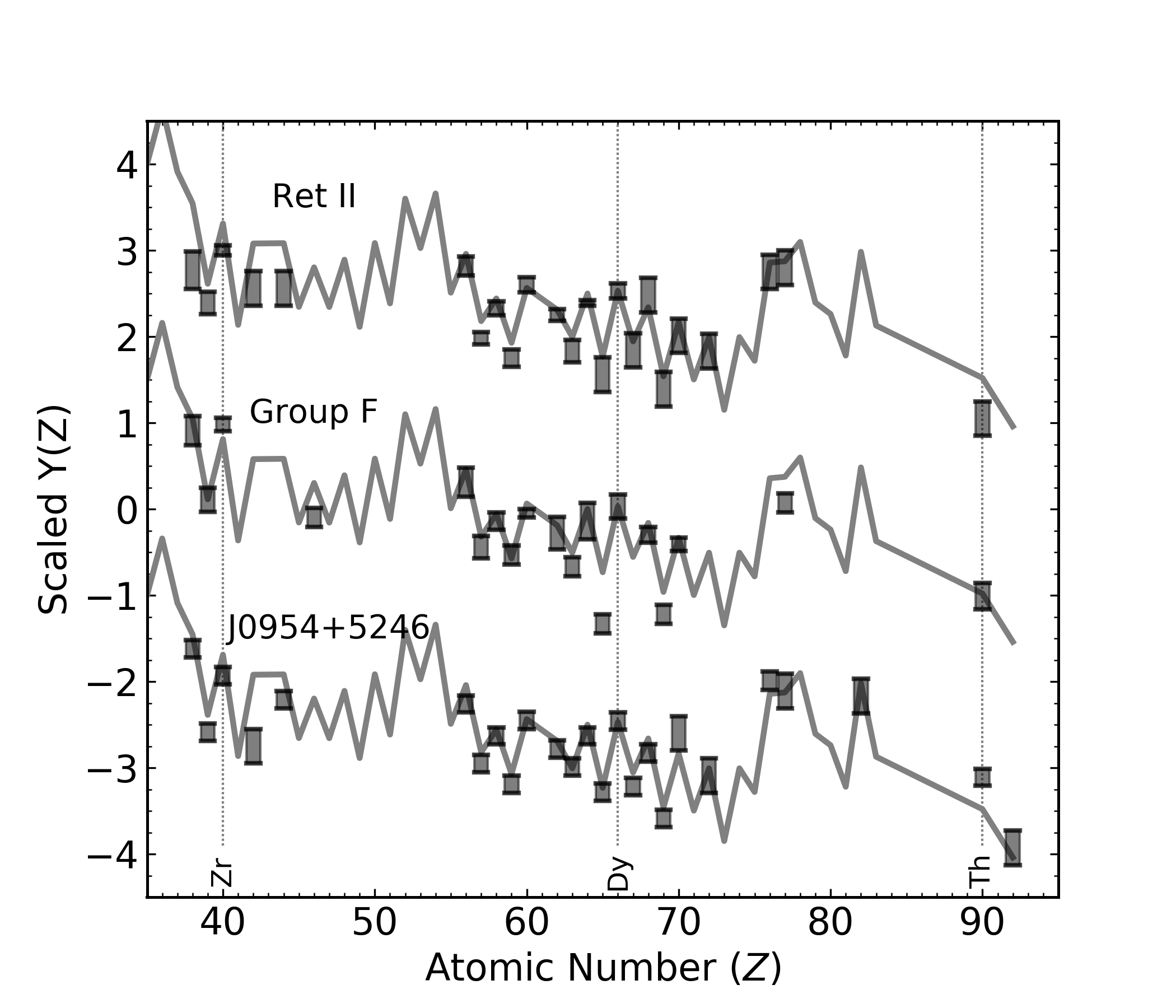# Actinide-Dilution with Matching model¶

Builds empircal mass ejecta distributions as a function of $Y_e$ (0.005-0.450)

To explain entire pattern from Zr to U

# ADM abundance pattern for Ret II¶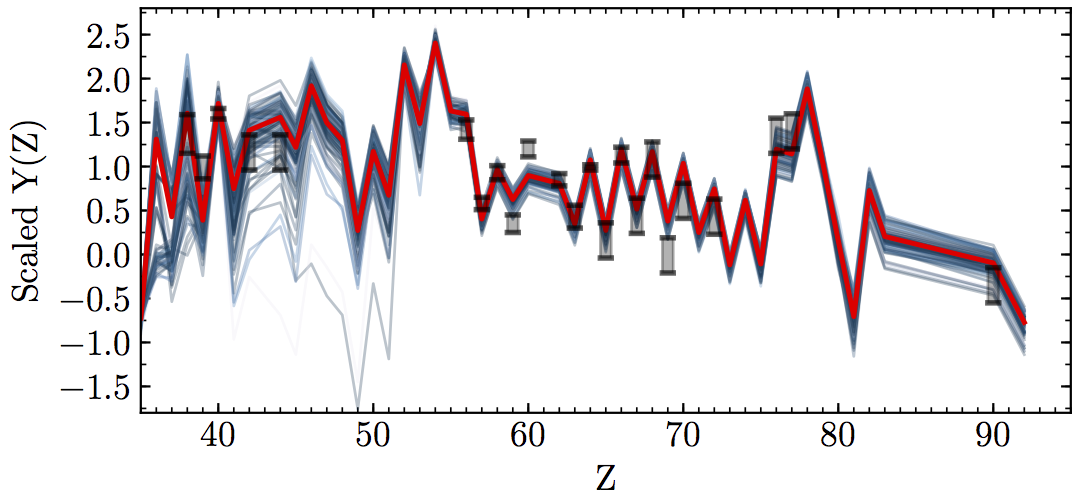# Empirically built ejecta mass distributions¶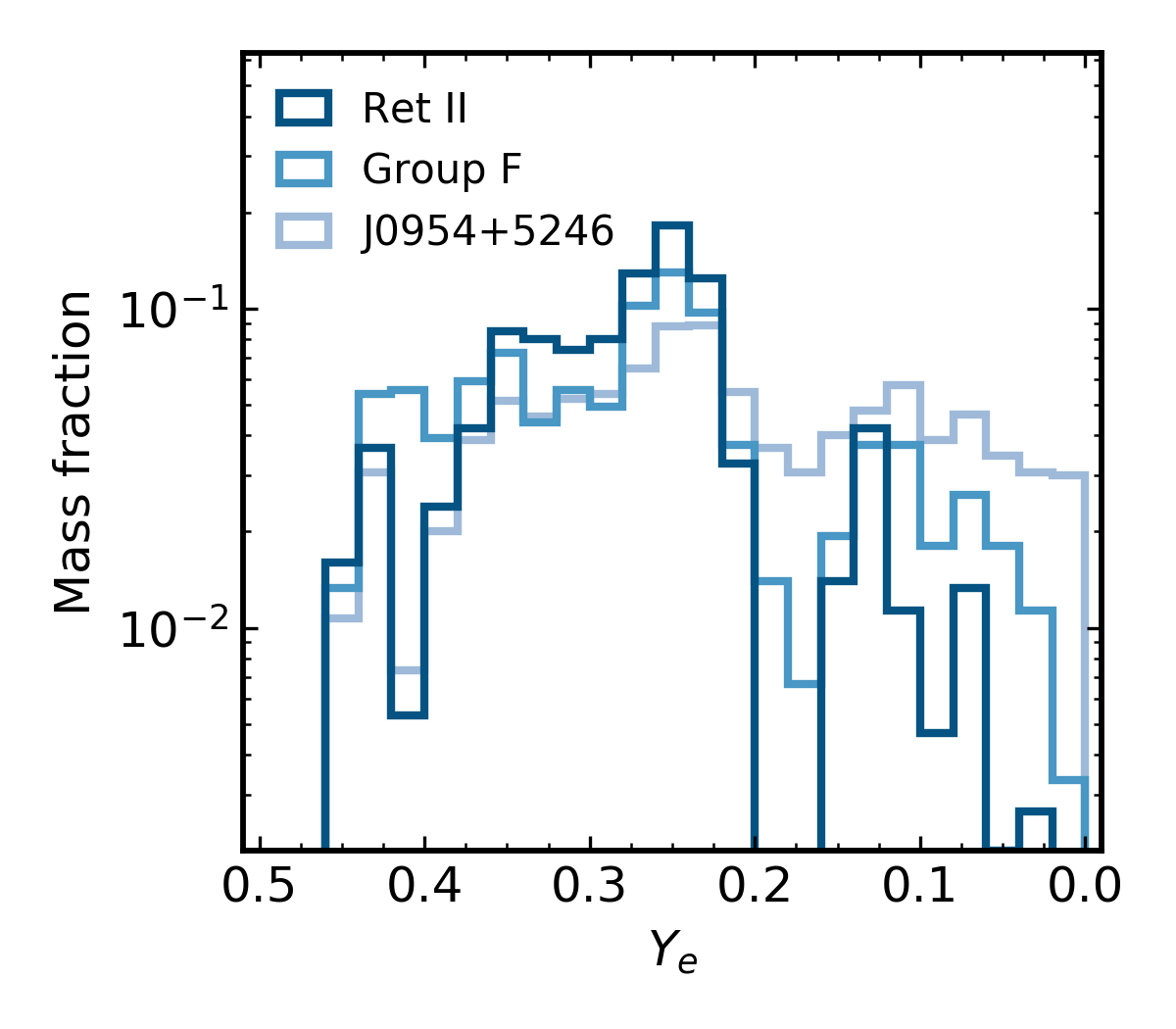## NSM ejecta (literature)¶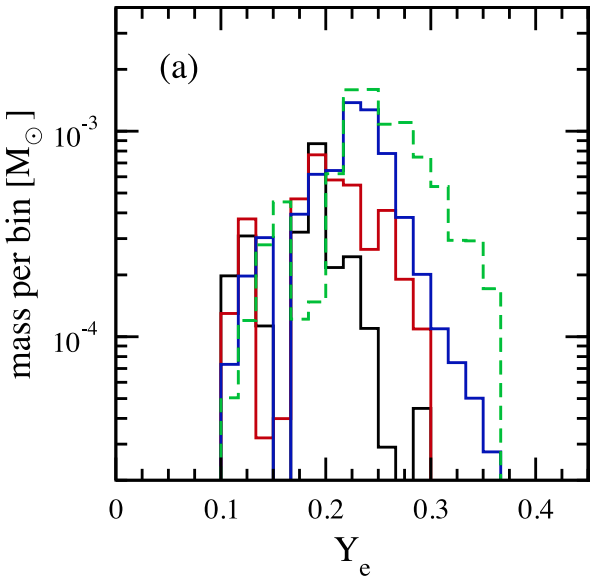# The low-Ye component¶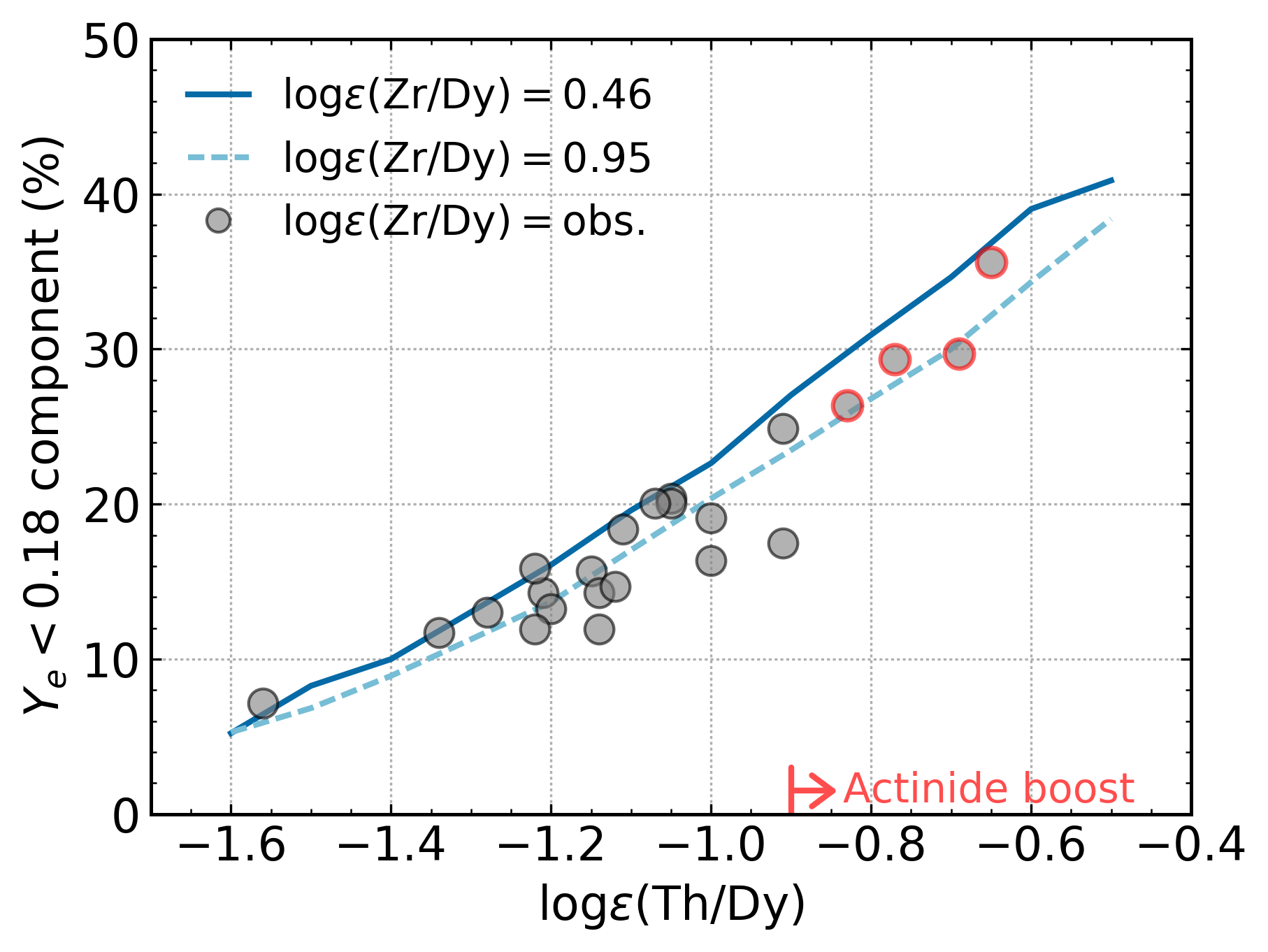# Low-Ye component under variations¶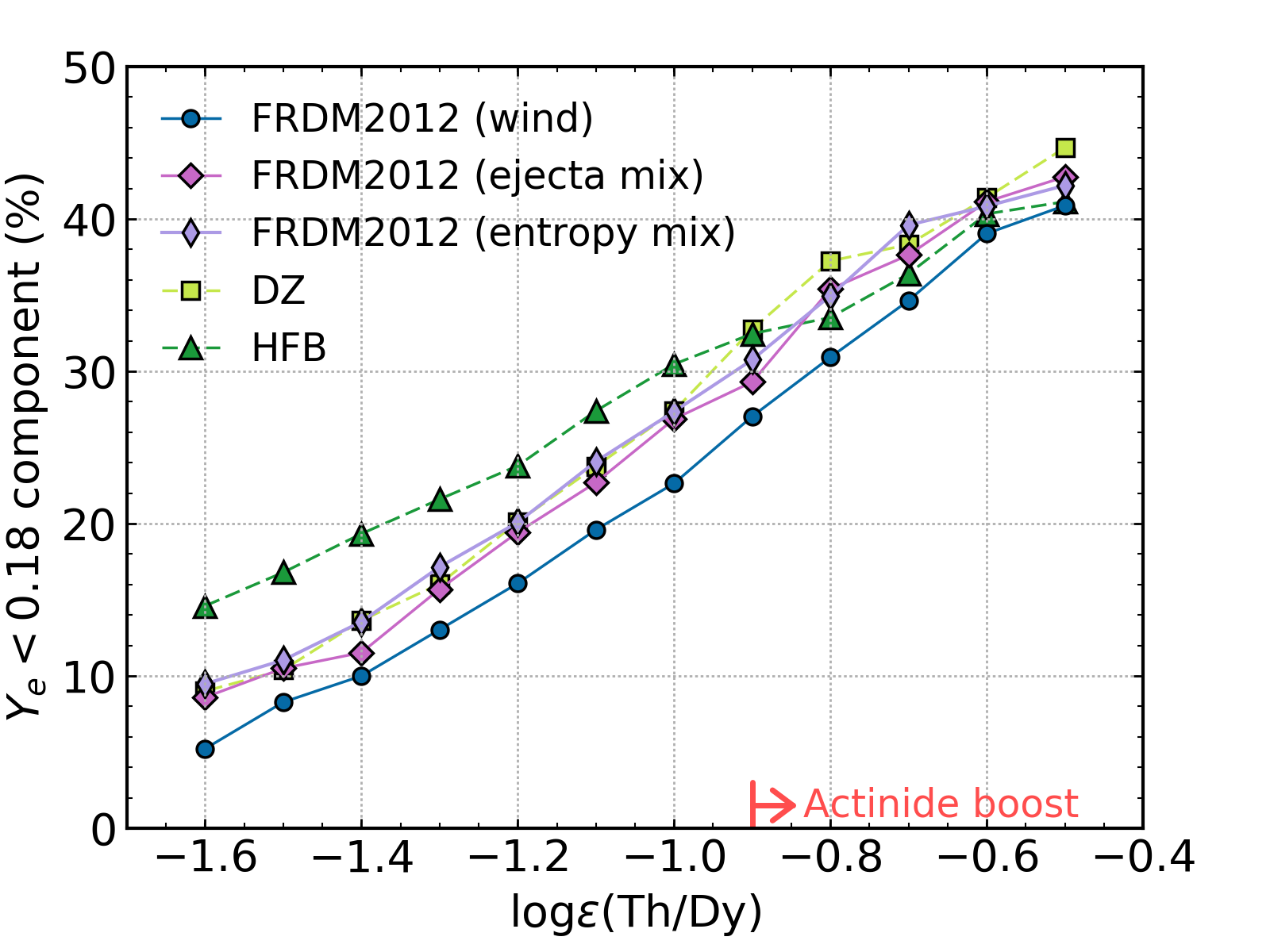# Actinide-boost stars do not call for a separate r-process progenitor

## Is this source an NSM?¶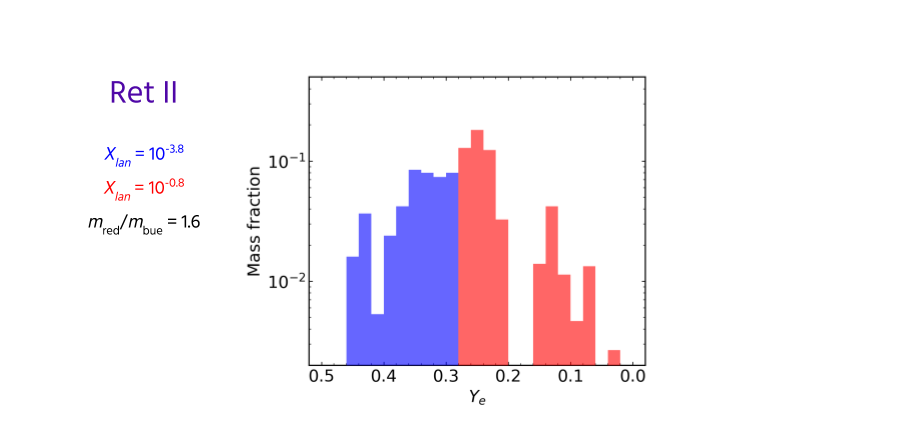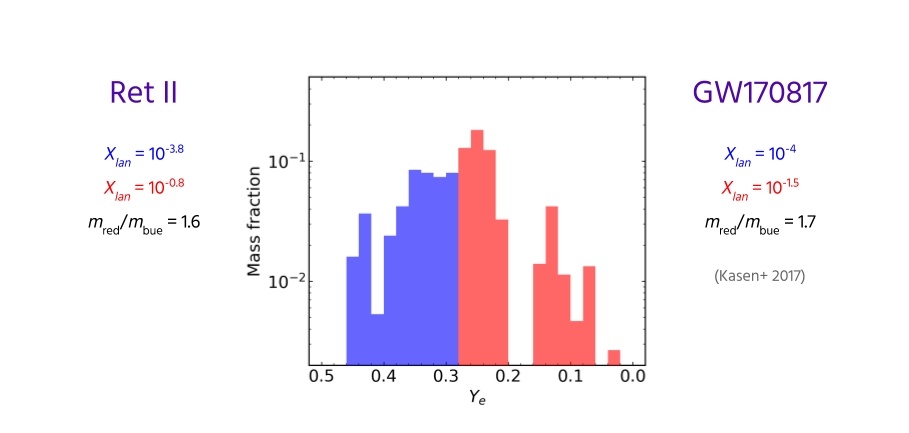# Summary and Outlook¶

The actinides are over-produced in very cold, neutron-rich (tidal) ejecta

NSMs could still be an actinide-boost source if most of the ejecta mass does not contribute actinides

The same r-process source can in principle account for observed actinide variations

How do the empirically built ejecta distributions compare to NSM simulations?

Entropy, dynamical timescale, nuclear physics variations...

# Special Thanks¶

Rebecca Surman (ND), Nicole Vassh (ND), Matthew Mumpower (LANL), Trevor M. Sprouse (ND)
Gail C. McLaughlin (NC State), Anna Frebel (MIT)

Timothy C. Beers (ND), Terese T. Hansen (TAMU), Chris Sneden (UT-Austin), Vinicius M. Placco (ND),
Ian U. Roederer (UMich.), Charli M. Sakari (UW), Rana Ezzeddine (MIT)
Grant Mathews (ND), Ani Aprahamian (ND), Toshihiko Kawano (LANL)